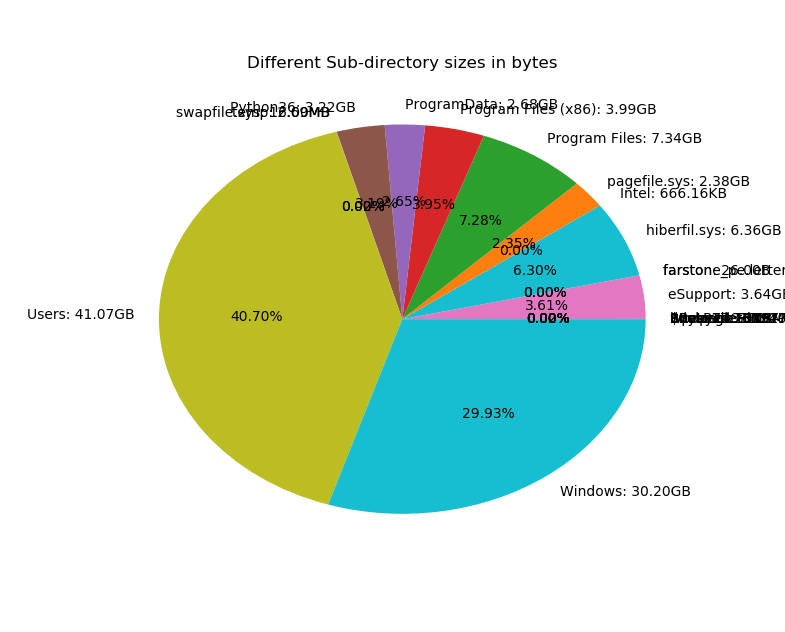# How to Get the Size of Directories in Python

Calculating the size of a directory in bytes in Python and plotting a pie using matplotlib to see which subdirectory takes most size.
4 min read · Updated may 2022 · Python Standard Library

Want to code faster? Our Python Code Generator lets you create Python scripts with just a few clicks. Try it now!

Disclosure: This post may contain affiliate links, meaning when you click the links and make a purchase, we receive a commission.

Have you ever wondered how you can get folder size in bytes using Python? As you may already know, os.path.get_size() function only returns the correct size of proper files and not folders. In this quick tutorial, you will learn how you can make a simple function to calculate the total size of a directory in Python.

Let's get started, open up a new Python file:

``import os``

The below core function calculates the total size of a directory given its relative or absolute path:

``````def get_directory_size(directory):
"""Returns the `directory` size in bytes."""
total = 0
try:
# print("[+] Getting the size of", directory)
for entry in os.scandir(directory):
if entry.is_file():
# if it's a file, use stat() function
total += entry.stat().st_size
elif entry.is_dir():
# if it's a directory, recursively call this function
try:
total += get_directory_size(entry.path)
except FileNotFoundError:
pass
# if `directory` isn't a directory, get the file size then
return os.path.getsize(directory)
except PermissionError:
# if for whatever reason we can't open the folder, return 0
return 0

Notice that I used the os.scandir() function which returns an iterator of entries (files or directories) in the directory given.

os.scandir() raises NotADirectoryError if the given path isn't a folder (a file or link), that's why we caught that exception and we return only the actual size of that file.

It also raises PermissionError if it cannot open the file (such as system files), in that case, we'll just return 0.

The above function will return the size in bytes, which will be of course, unreadable for large directories, as a result, let's make a function to scale these bytes to Kilo, Mega, Giga, etc:

``````def get_size_format(b, factor=1024, suffix="B"):
"""
Scale bytes to its proper byte format
e.g:
1253656 => '1.20MB'
1253656678 => '1.17GB'
"""
for unit in ["", "K", "M", "G", "T", "P", "E", "Z"]:
if b < factor:
return f"{b:.2f}{unit}{suffix}"
b /= factor
return f"{b:.2f}Y{suffix}"``````

Alright, I'm gonna test this on my C drive (I know it's large):

``get_size_format(get_directory_size("C:\\"))``

This took about a minute and returned the following:

``'100.91GB'``

Now, what if I want to know which subdirectories are taking most of this space? Well, the following code doesn't just calculate the size of each subdirectory, but plots a pie using matplotlib library (in which you can install using pip3 install matplotlib) that shows the size of each of them:

``````import matplotlib.pyplot as plt

def plot_pie(sizes, names):
"""Plots a pie where `sizes` is the wedge sizes and `names` """
plt.pie(sizes, labels=names, autopct=lambda pct: f"{pct:.2f}%")
plt.title("Different Sub-directory sizes in bytes")
plt.show()

if __name__ == "__main__":
import sys
folder_path = sys.argv

directory_sizes = []
names = []
# iterate over all the directories inside this path
for directory in os.listdir(folder_path):
directory = os.path.join(folder_path, directory)
# get the size of this directory (folder)
directory_size = get_directory_size(directory)
if directory_size == 0:
continue
directory_sizes.append(directory_size)
names.append(os.path.basename(directory) + ": " + get_size_format(directory_size))

print("[+] Total directory size:", get_size_format(sum(directory_sizes)))
plot_pie(directory_sizes, names)``````

Now, this takes the directory as an argument in the command line:

``python get_directory_size.py C:\``

This will show a nice pie that looks something like this:Now after seeing this chart, I know Users and Windows folders are taking most of my C drive!

Alright, this is it for this tutorial, If you want to learn more about handling files and directories in Python, check this tutorial.

We also have a tutorial for organizing files by extension in your machine, make sure to check it out as well!

Finally, if you're a beginner and want to learn Python, I suggest you take the Python For Everybody Coursera course, in which you'll learn a lot about Python, good luck!

Read also: How to List all Files and Directories in FTP Server using Python.

Happy Coding ♥

Just finished the article? Now, boost your next project with our Python Code Generator. Discover a faster, smarter way to code.

Sharing is caring!

### Comment panel

Got a coding query or need some guidance before you comment? Check out our Python Code Assistant for expert advice and handy tips. It's like having a coding tutor right in your fingertips!